### Choose language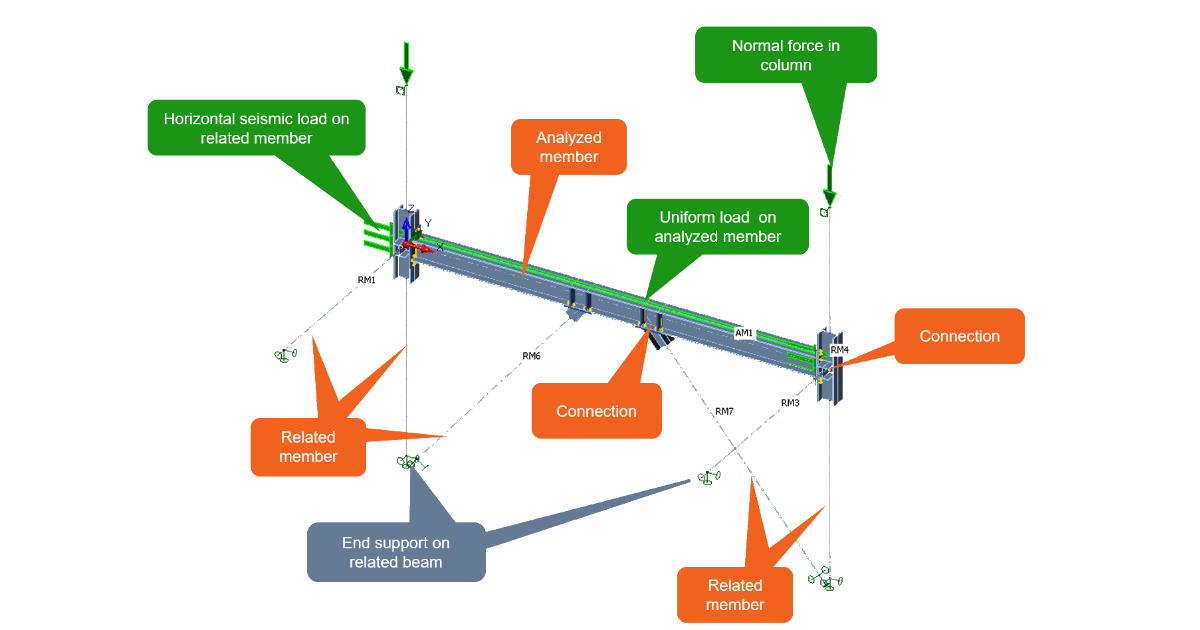# Member model organization

$$IDEA StatiCa Member model organization is slightly different than in the other IDEA StatiCa applications however it still respects the CBFEM approach. Let's go through the key definitions.Analyzed member is a part of the structure which is meant to be analyzed, the part in which we are interested in. Related members are all other members that are connected to the analyzed one. Related members have common joints with the analyzed one.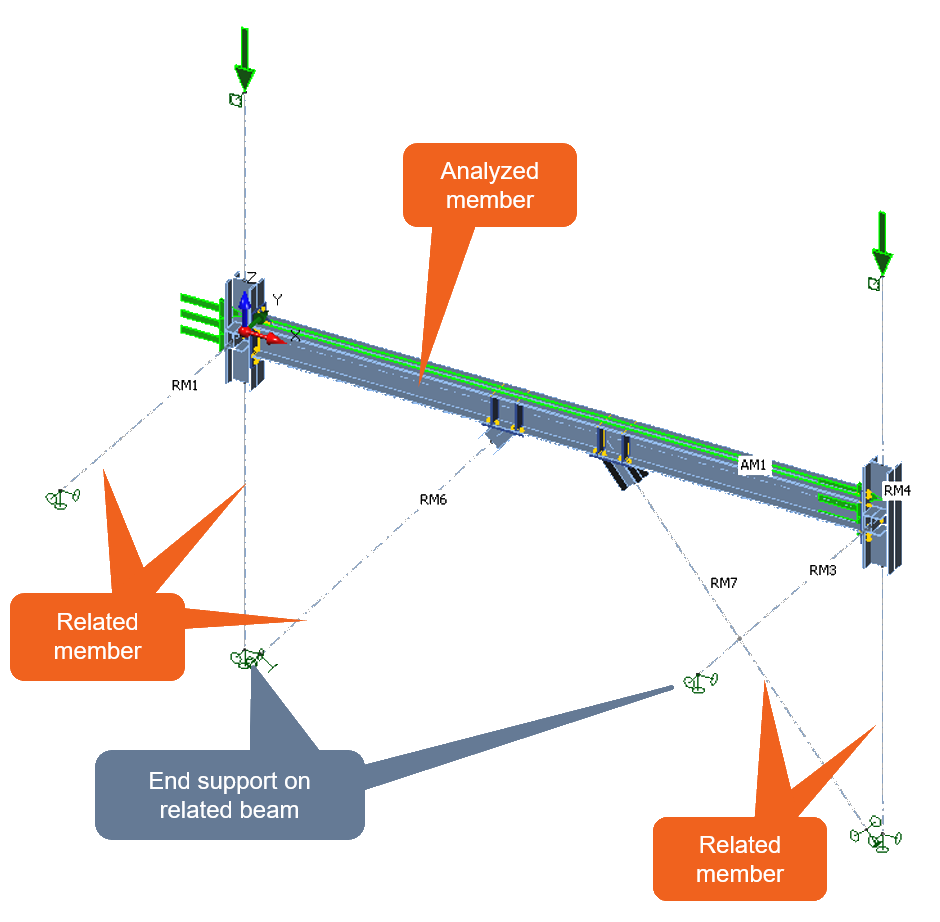Connections between the Analyzed member and the Related members are defined by the joint design.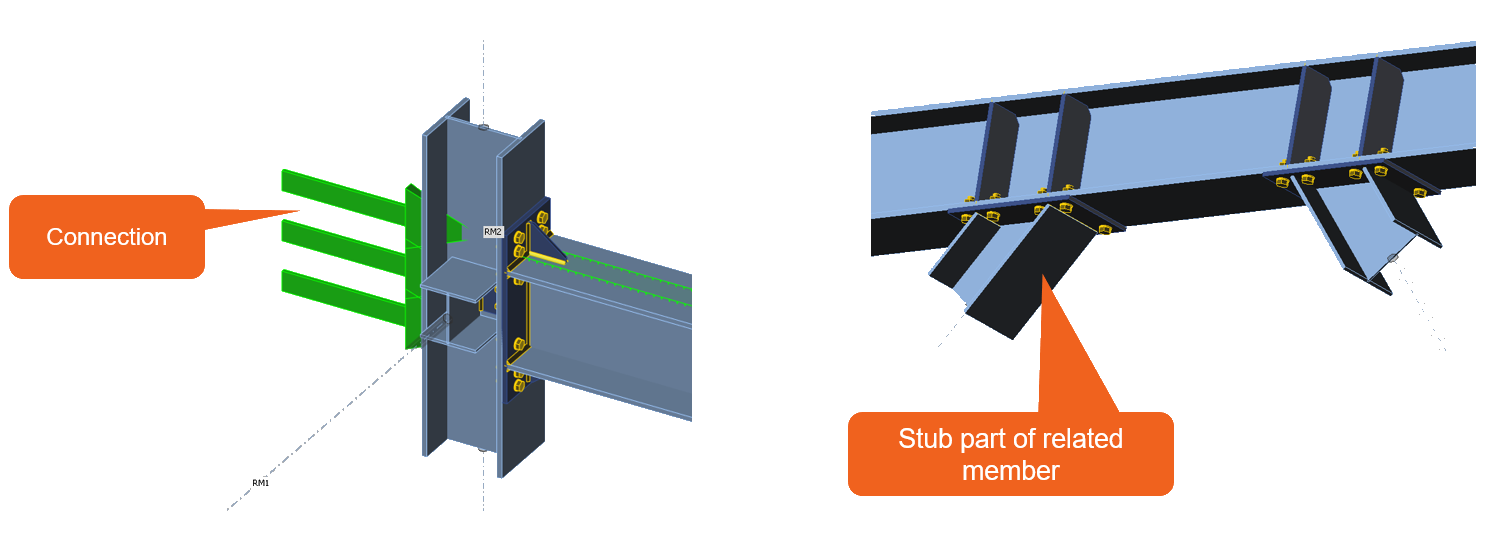Loads can be introduced in several ways. Basically two possibilities can be distinguished - Internal forces and applied loads. Internal forces can be introduced at the end of a related member. Applied loads can be introduced on the Analyzed member or on Related members as the uniform load.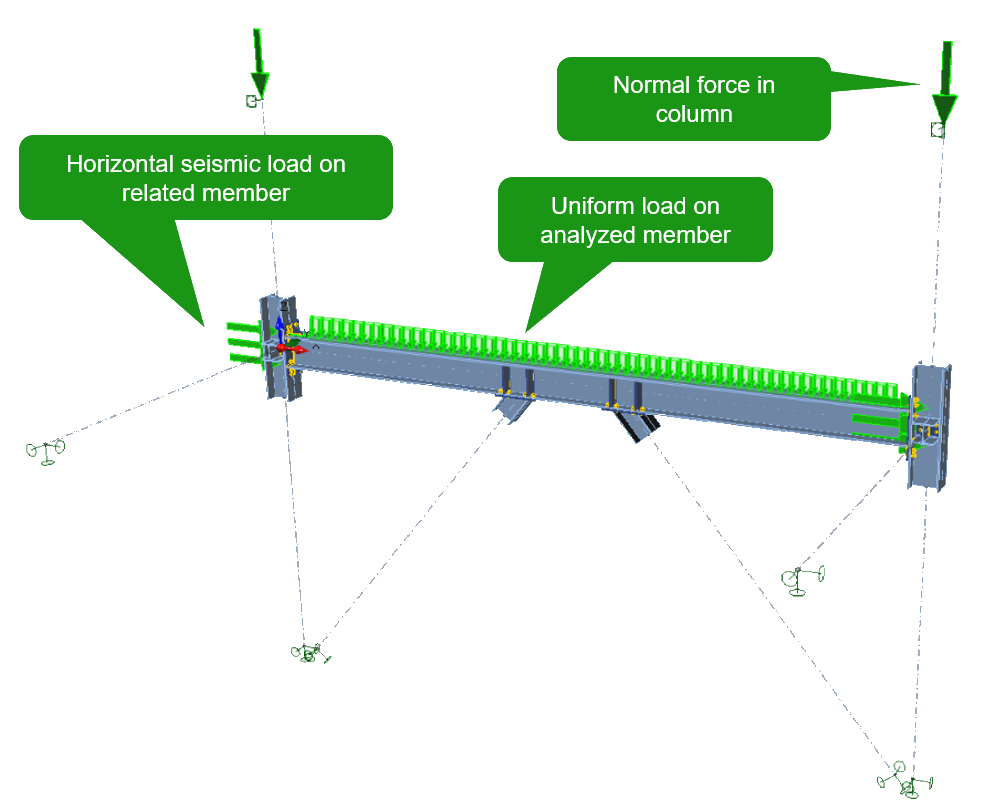By defining all these parts of the model, the Member organization is complete.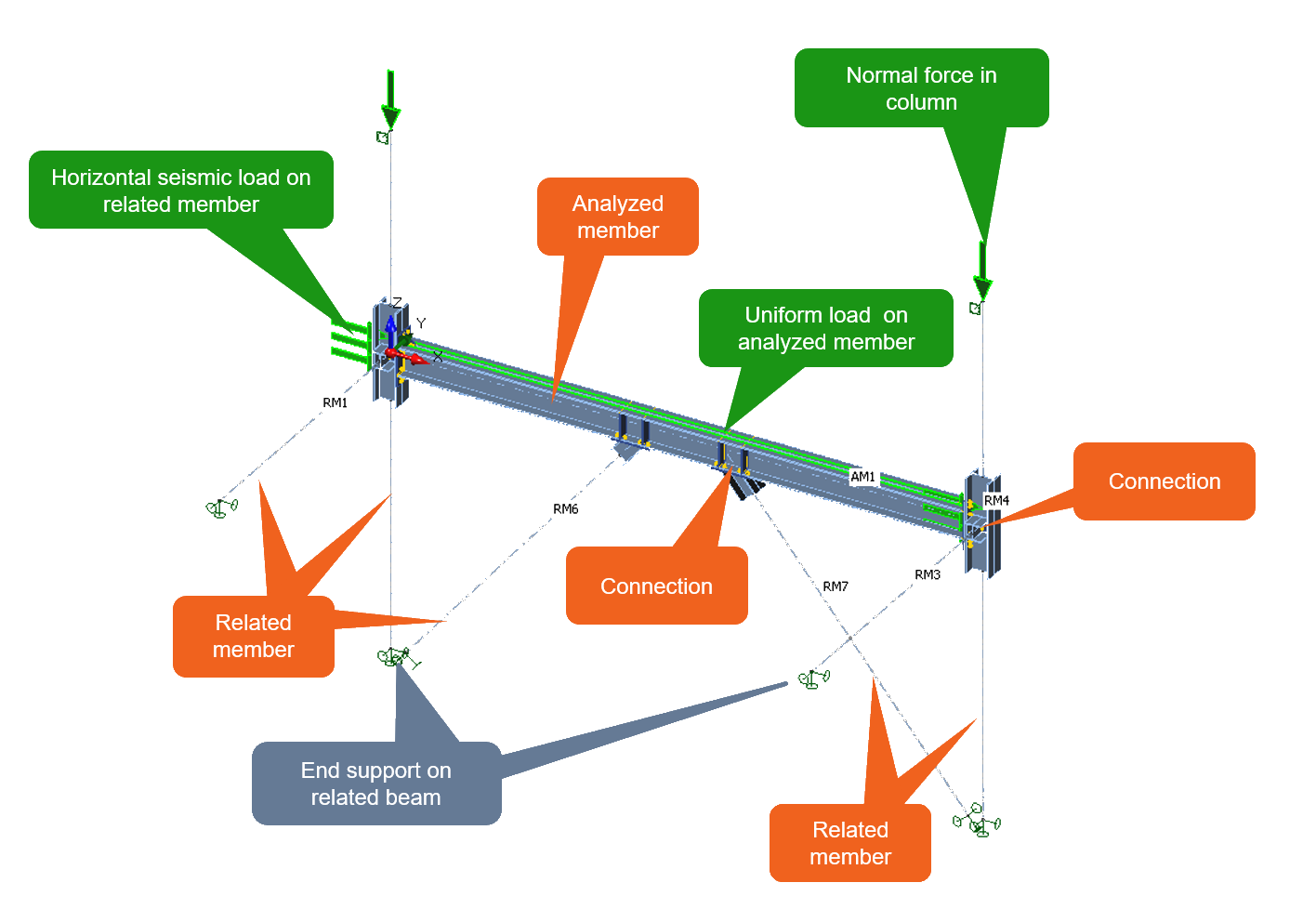The whole description of the model workflow is explained in the Theoretical background for IDEA StatiCa Member.$$